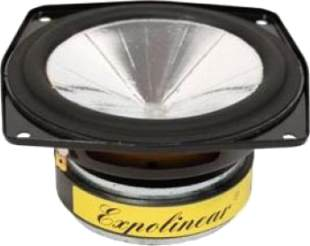# Görlich

## Midsrecommended cabinet 1:
closed cabinet with 2 L volume
from 118/75 Hz (-3dB/-8dB)

recommended cabinet 2:
4,3 L volume bass reflex cabinet
with HP35 reflex tube, 15 cm long.
from 74/55 Hz (-3dB/-8dB).

## Görlich TT130G

order no. E-goe-tt130g

- not available (_)

12 cm midrange driver with aluminium cone. To avoid compression effects the driver possesses a pole piece hole.

• power handling (continuous/programme) = 50/60 W
• resonance frequency fs = 33 Hz
• impedance R = 8 Ohm
• sound pressure level SPL = 86 dB (2,83V; 1m)
• DC resistance Re = 6,5 Ohm
• force factor BL = 6,3 N/A
• voice coil inductance L = 0,71 mH
• effective piston radiating area Sd = 82 cm2
• effective mechanical mass incl. air load mms = 7,7 g
• equivalent volume of compliance Vas = 29 l
• total Q factor Qts = 0,23 (Qms=1,7, Qes=0,26)
• voice coil diameter = 25 mm
• mounting diameter d = 110 mm
• overall diameter d = 131 mm
• mounting depth (not countersunk) t = 62 mm
PDF data sheetrecommended cabinet 1:
closed cabinet with 9,9 L volume
from 76/48 Hz (-3dB/-8dB)

recommended cabinet 2:
23 L volume bass reflex cabinet
with HP70 reflex tube, 27 cm long.
from 46/34 Hz (-3dB/-8dB).

## Görlich TT170G

order no. E-goe-tt170g

- not available (_)

16 cm midrange driver with aluminium cone. To avoid compression effects the driver possesses a pole piece hole.

• power handling (continuous/programme) = 60/70 W
• resonance frequency fs = 23 Hz
• impedance R = 8 Ohm
• sound pressure level SPL = 87 dB (2,83V; 1m)
• DC resistance Re = 7 Ohm
• force factor BL = 7 N/A
• voice coil inductance L = 0,82 mH
• effective piston radiating area Sd = 154 cm2
• effective mechanical mass incl. air load mms = 13 g
• equivalent volume of compliance Vas = 117 l
• total Q factor Qts = 0,25 (Qms=2, Qes=0,28)
• voice coil diameter = 30 mm
• mounting diameter d = 155 mm
• overall diameter d = 176 mm
• mounting depth (not countersunk) t = 80 mm
PDF data sheet## Görlich TT200G

order no. E-goe-tt200g

- not available (_)

bass driver with aluminium cone. To avoid compression effects the driver possesses a pole piece hole.

• resonance frequency fs = 32 Hz
• impedance R = 8 Ohm
• DC resistance Re = 6,9 Ohm
• force factor BL = 10,5 N/A
• voice coil inductance L = 1,3 mH
• effective mechanical mass incl. air load mms = 20 g
• equivalent volume of compliance Vas = 57,7 l
• total Q factor Qts = 0,22 (Qms=1,8, Qes=0,25)
• voice coil diameter = 30,2 mm
• mounting diameter d = 182 mm
• mounting depth (not countersunk) t = 95 mm
PDF data sheet

The cabinet volume calculations are executed considering an external resistance of 0.3 Ohm. We are using, if no other data is known or available, the information supplied by the manufacturer.

Please note: the frequency response doesn't say much about the cut-off frequency inside the cabinet! Therefore, we mostly give you 2 examples with dimensioning considerations and an indication, how low the loudspeaker will reproduce.

Home